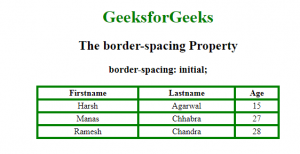Open In App

# CSS border-spacing Property

The border-spacing Property is used to set the distance between the borders of neighboring cells in the Table. This property works only when the border-collapse property is set to no-collapse separate.

Default Value:

• 2px

Syntax:

`border-spacing: length|initial|inherit; `

Property values:

1. length-length: It is used to set the distance between the borders of adjacent cells. It does not allow negative values.
• If two values are defined, first value defines the horizontal space and second value defines the vertical spacing.
• If only one value is given then it defines the both horizontal and vertical spacing between the adjacent borders of cells.
2. initial: It sets the property to its default value.

Syntax:

`border-spacing:initial;`

Example:

## html

 ```<``html``>` `<``head``>``    ``<``title``>border-spacing property``    ``<``style``>``        ``table,``        ``th,``        ``td {``            ``border: 2px solid green;``            ``text-align: center;``        ``}``        ` `        ``#geeks {``            ``border-collapse: separate;``            ``background-color: none;``            ``border-spacing: initial;``        ``}``        ` `        ``h1 {``            ``color: green;``        ``}``    ````` `<``body``>``    ``<``center``>``        ``<``h1``>GeeksforGeeks``        ``<``h2``> The border-spacing Property``        ``<``h3``>border-spacing: initial;``        ``<``table` `style``=``"width:70%"` `id``=``"geeks"``>``            ``<``tr``>``                ``<``th``>Firstname``                ``<``th``>Lastname``                ``<``th``>Age``            ````            ``<``tr``>``                ``<``td``>Harsh``                ``<``td``>Agarwal``                ``<``td``>15``            ````            ``<``tr``>``                ``<``td``>Manas``                ``<``td``>Chhabra``                ``<``td``>27``            ````            ``<``tr``>``                ``<``td``>Ramesh``                ``<``td``>Chandra``                ``<``td``>28``            ````        ````    ````` `                  `

Output:Supported Browsers: The browser supported by css border-spacing property are listed below: